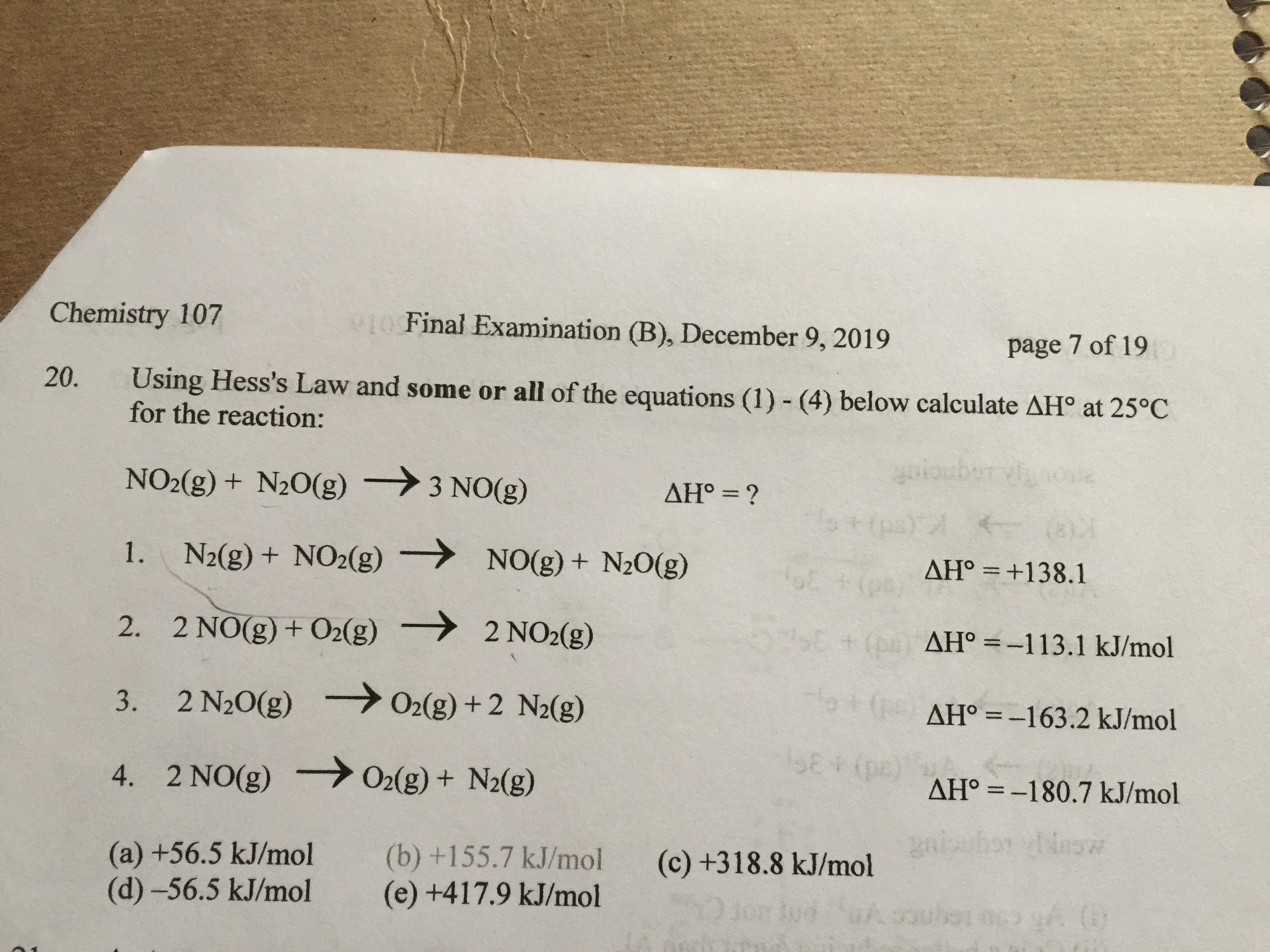# Using Hess's Law and some or all of the equations (1) - (4) below calculate ΔH° at 25°C for the reaction: NO2(g) + N2O(g) → 3 NO(g). 1. N2(s) + NO2(g) → NO(g) + NO2(g) ΔH1 = +138.1 kJ/mol 2. 2 NO(g) + O2(g) → 2 NO2(g) ΔH2 = –113.1 kJ/mol 3. 2 N2O(g) → O2(g) + 2 N2(g) ΔH3 = –163.2 kJ/mol 4. 2 NO(g) → O2(g) + N2(g) ΔH4 = –180.7 kJ/mol